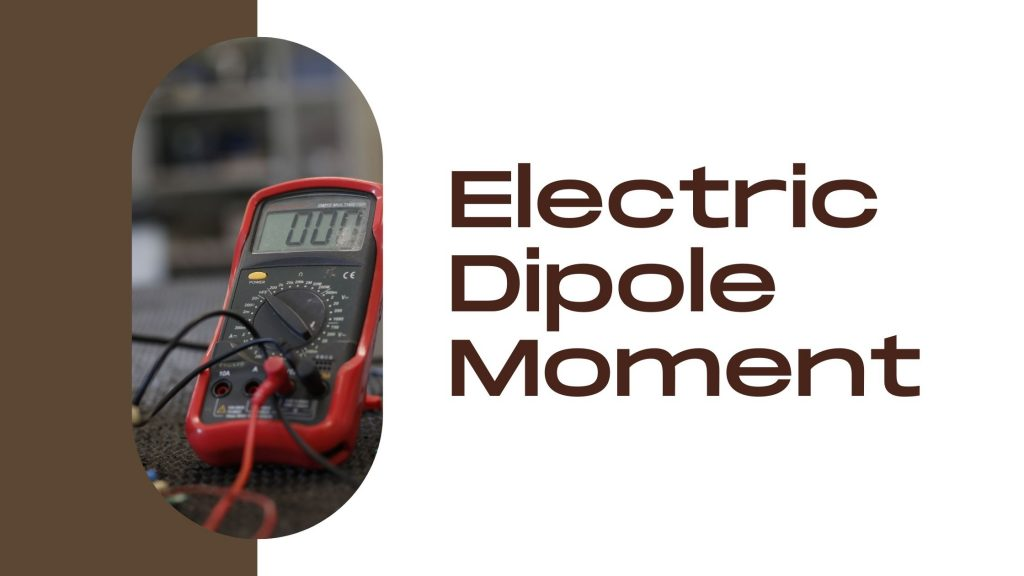Physics
Study Material

# Electric Dipole Moment- Definition, Unit, Formula, Electric Field

The electric dipole moment is the product of the magnitude of the charge and the distance between the centres of positive (+q) and negative charges (-q). Read in detail below.

7 minutes long
Posted by Mahak Jain, 20/10/2021Hesap Oluştur

Got stuck on homework? Get your step-by-step solutions from real tutors in minutes! 24/7. Unlimited.

An electric dipole is a pair of equal and opposite point charges q and –q, separated by a distance 2a.  Example : two equal charge +q and -q separated by distance 2a is form a dipole. In an electric dipole, the magnitude of both the charges will be the same, a pair of charges with two different magnitude can not be considered as dipole. Since, both the charges are equal and opposite in charge therefore the net charge is zero. ie : (+q) + (-q) = zero. Since, the net charge is zero this does not mean that the field of the electric dipole is zero. Thus, producing electric dipole moment.

## What is Electric Dipole Moment ?

The electric dipole moment is a measure of the separation of positive and negative electrical charges within a system. As it is the product of magnitude of charge and distance therefore it determines the strength of an electric dipole to produce the electric field. It measures the polarity of system consisting two opposite and equal charges.

Dipole moment(p) = magnitude of charge(q) × distance (d)

The dipole moment and distance between both charges are the vector quantity therefore the dipole moment is a vector quantity and denoted by P.

## Define Electric Dipole Moment

The electric dipole moment is the product of the magnitude of the charge and the distance between the centres of positive (+q) and negative charges (-q).

Two equal charge +q and -q are separated by distance 2a.

Dipole moment = magnitude of charge × distance between charge

Then the dipole moment is,

P = q × 2a

Where,

q – The magnitude of the charge

2a – The separation between two charges

## SI Unit of Electric Dipole Moment

As electrical dipole moment is product of  magnitude of charge and distance separating two charges.

SI unit of charge (q) is coulomb and SI unit of distance Is meter.

Since, dipole moment p = q × 2a

So, P  = coulomb × meter

Therefore, SI unit of electric dipole moment is coulomb-meter (Cm).

## Direction of Electric Dipole Moment

The electric dipole moment is a vector quantity therefore it has a defined direction. The direction of the electric dipole moment from negative charge to positive charge. The line along the direction of electric dipole moment is known as axis of the dipole moment.

## Formula of Electric Dipole Moment

Dipole moment is product of magnitude of charge and distance separating both charges.

Dipole moment = Magnitude of charge × distance of separation

P = q × d

Here, P = dipole moment, q = charge, d = separating distance

## Electric Field Due to a Dipole

The electric field of the pair of charges at any point in space can be found out from Coulomb’s law and the superposition principle.

• For points on the axis

Point P be at distance r from the centre of the dipole on the side of the charge q as

Then electric field due to charge -q on point P is,

Electric field due to charge +q on point P is,

Thus, the total field at point P is,

Etotal = E+q + E-q

• For points on the equatorial plane

Point is in equatorial plan to dipole as,

Electric field due to -q is, E-q = q / 4πε (r² + a²)

Electric field due to +q is, Eq = q / 4πε (r² + a²)

Now point P is at angle θ from both the charges the components normal to the dipole axis cancel away due to equal and opposite direction . The components along the dipole axis add up as

At large distances, r >> a electric field is ,

## Electric Potential Due To Dipole

The electric potential is the amount of work energy needed to move a unit of electric charge from a one point to the specific point in an electric field.

The electric potential due to a point charge q at a distance of r from that charge is

V = q / 4πε0 × r

Now total electric potential due to both the +q and -q charges are,

Thus, V = q / 4πε0 (r2-r1 / r1r2 )

Here, r1 = distance between +q to point p and r2 = distance between -q to point p

When r is greater than a (r >> a) then

r1 ≈ r ≈ r2

by drawing a line perpendicular to r 2 from +q we can write

r2 – r1 ≈ 2a cosθ

Now Electric potential V at point P is ,

## Torque on a Dipole in Uniform Electric Field

A dipole of dipole moment p in a uniform external field E ,

Now due to electric field there is qE force on +q charge and -qE force on -q charge. Since both the forces are working in opposite direction therefore it produces a torque on the dipole. The magnitude of this torque is equal to the magnitude of each force multiplied by the arm of the couple (perpendicular distance between the two antiparallel forces).

Magnitude of torque, τ = q E × 2 a sinθ

Thus, τ = 2qa Esinθ

Hence, Torque = τ = pE sinθ         (Since q × 2a = p )

Torque = p × E, where p and E are in vector form.

Thus a dipole in a uniform electric field experiences a torque.